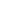| lesson material | material summary | questions and answers | definitions | types and examples | other information | materi pelajaran | ringkasan materi | pertanyaan dan jawaban | definisi | jenis-jenis dan contoh-contoh | informasi lainnya |

# Learning Javascript: Knowing Math Objects for Mathematical CalculationsHave you ever made a program in which there were mathematical calculations?
For example, such as calculating square roots, rank, log, sin, cos, tan, and others.
This can be made with operators and several variables .
But it's not easy ...
Because, we have to think about the algorithm for ourselves.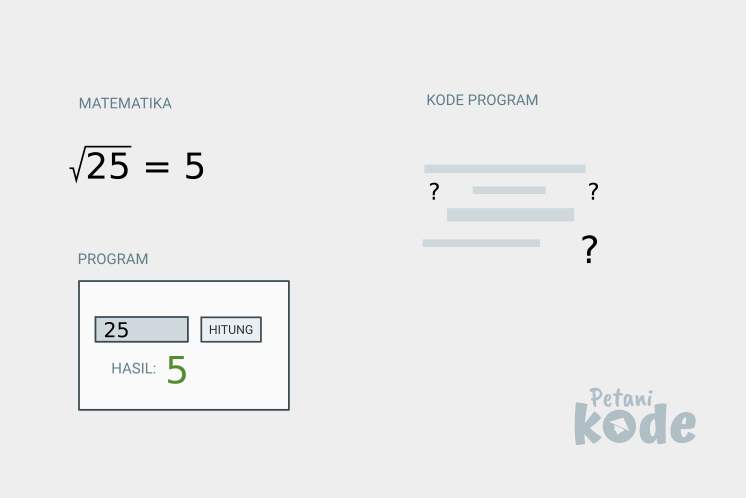But take it easy, there is an object in Javascript `Math` that will help us make mathematical calculations.

## Know the Math Object

Objects `Math`are objects that contain mathematical functions and some constants to do mathematical calculations such as sin, cos, tan, exponent, square root, etc.
So that it is easier to understand the contents of an object `Math`, we divide it into several groups like this: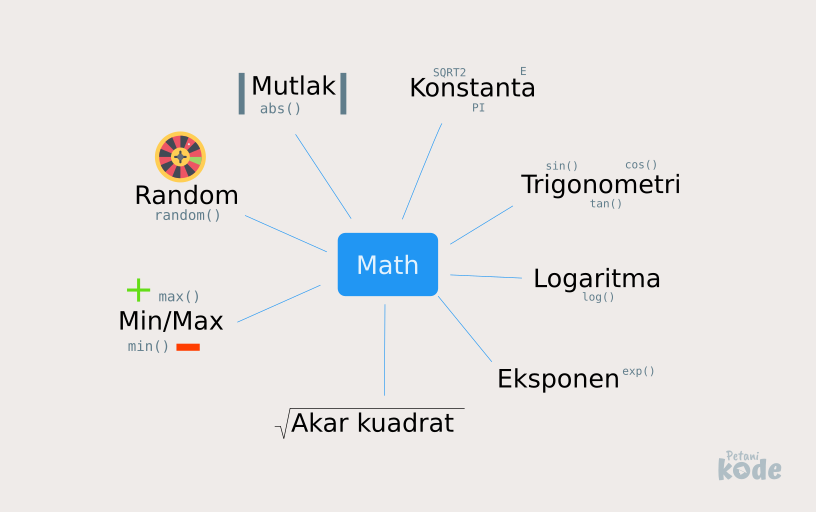These are functions commonly used in mathematical calculations.
OK…
Let's discuss more about each of these functions.
First we start from:

## Trigonometry function in Javascript

Trigonometry is a branch of mathematics that studies angles and lengths in triangles. 1
In the computer world, this knowledge is usually implemented in computer graphics.
You may have read game-making tutorials in Python .
There we use trigonometry to trune pointer movements.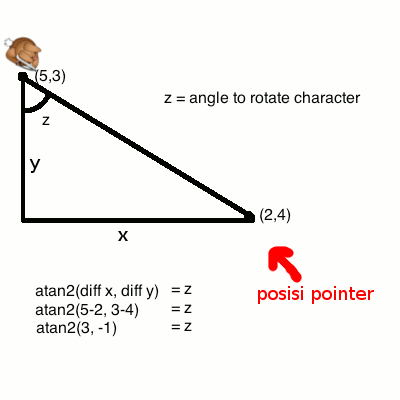Well, in objects `Math`there are functions for calculating trigonometry.
Suppose we want to calculate the sin value from `10`, then in the program we can write it like this:
``````var n = Math.sin(10);
``````
The variable `n`will contain `-0.5440211108893698`because sin `10` is `0.5`.
You can try it yourself through the Javascript console: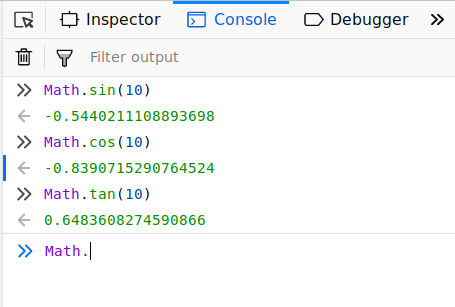## Logarithmic, Rank, and Exponential Functions in Javascript

Logarithms are mathematical operations which are the inverse of exponents or lifts. 2
The object `Math`in Javascript also provides functions `log()` for logarithms and `pow()`for appointment.
Let's try on the console: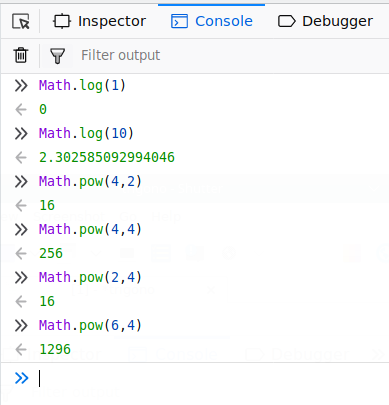Then to calculate exponential, we can use the function `exp()`.
Example: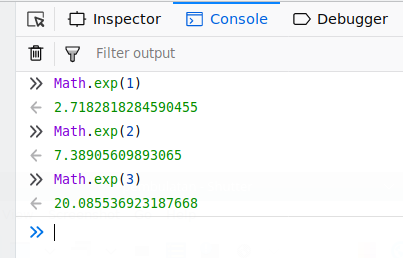## Rounding function in Javascript

If we need integers (integer) , we can gungakan pembulatang function in the object `Math`.
There are several functions that are often used:
1. `floor()` round down;
2. `round()` round to the closest, can go down and up;
3. `ceil()` round up.
Example: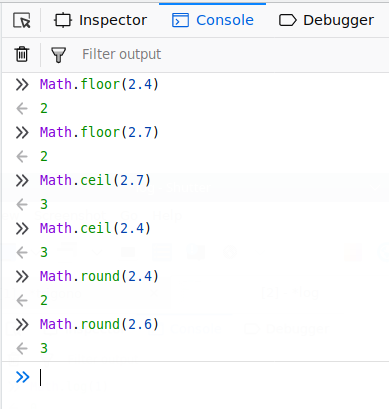## Root function in Javascript

Well, if the square root function ...
We can calculate it with functions `sqrt()`.
Example: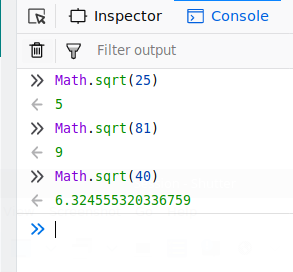Then what about the cubic root?
Easy…
We can use the function `cbrt()`.
Example: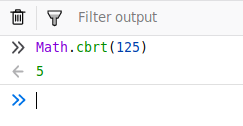Now for root n or root, we can use the function `pow()`.
Example:
``````Math.pow(n, 1/root);
``````
`n`is the value we are looking for, then `root`is the root n.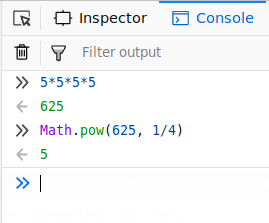## Random and Absolute Functions in Javascript

A random function is a function that makes it impossible for random values `0.0`to arrive at `1.0`.
Example: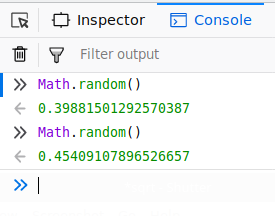If you want to make a random value from a certain range of values, then we can use the help function `floor()`to round up and multiply by the value of min and max.
The formula will be like this:
``````Math.floor(Math.random() * (max - min) ) + min;
``````
We can wrap this in functions like this:
``````function getRndInteger(min, max) {
return Math.floor(Math.random() * (max - min) ) + min;
}
``````
The result: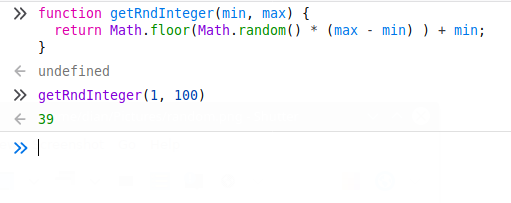Next we discuss the absolute function.
Absolute function is a function that generates an absolute value or absolute .
Example:
``````var x = Math.abs(-2)
``````
Variables `x`will be valuable `2`, because the function `abs()` will always provide absolute or positive values.

## Minimum and Maximum Functions in Javascript

The minimum and maximum functions are functions to determine the smallest and largest value in a set of values.
We can give this function input in the form of a number.
If we want to provide an input array, we have to break the array of contents.
Example: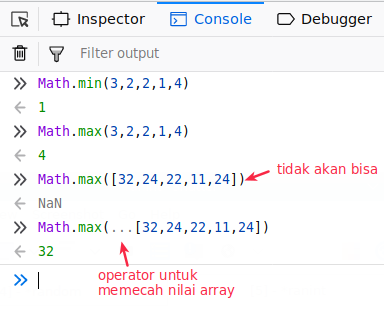Pay attention to the operator `...`, this is the operator added to the ES2015 which functions to break the contents of arrays and strings.

## Constants in the Math Object

In addition to providing mathematical functions, objects `Math`also provide constants such as `PI``E``LN10`, etc. which we can use for calculating certain formulas. 3
``````Math.E        // returns Euler's number
Math.PI       // returns PI
Math.SQRT2    // returns the square root of 2
Math.SQRT1_2  // returns the square root of 1/2
Math.LN2      // returns the natural logarithm of 2
Math.LN10     // returns the natural logarithm of 10
Math.LOG2E    // returns base 2 logarithm of E
Math.LOG10E   // returns base 10 logarithm of E ``````
Reference: https://www.petanikode.com/javascript-matematika/
##### 0 Komentar untuk "Learning Javascript: Knowing Math Objects for Mathematical Calculations"

Silahkan berkomentar sesuai artikel

Template By Kunci Dunia